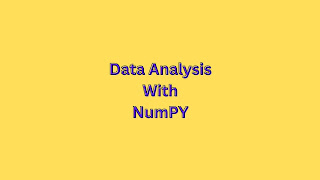### The Quick and Easy Way to Analyze Numpy ArraysThe quickest and easiest way to analyze NumPy arrays is by using the numpy.array() method. This method allows you to quickly and easily analyze the values contained in a numpy array. This method can also be used to find the sum, mean, standard deviation, max, min, and other useful analysis of the value contained within a numpy array. Sum You can find the sum of Numpy arrays using the np.sum() function.  For example:  import numpy as np  a = np.array([1,2,3,4,5])  b = np.array([6,7,8,9,10])  result = np.sum([a,b])  print(result)  # Output will be 55 Mean You can find the mean of a Numpy array using the np.mean() function. This function takes in an array as an argument and returns the mean of all the values in the array.  For example, the mean of a Numpy array of [1,2,3,4,5] would be  result = np.mean([1,2,3,4,5])  print(result)  #Output: 3.0 Standard Deviation To find the standard deviation of a Numpy array, you can use the NumPy std() function. This function takes in an array as a par

JavaScript Object Notation (JSON) was invented by Douglas Crockford as a subset of JavaScript syntax to be a lightweight data format that is easily readable and writable by both humans and machines. In general, JSON is considered terse when compared to other interchange formats.

After you become familiar with JSON, you will find it fairly easy to read complex JSON data structures. Even though JSON is based on a subset of the JavaScript programming language, it is considered language independent.

## JSON XML

The flexibility of XML has made it increasingly prevalent in programming environments. Unlike the Unix® world, where configuration files are usually text files with either tab-delimited name/value pairs or colon-separated fields, configuration files in the open source world are often XML documents.

Most well-known application servers also use XML-based configuration files. The Ant utility relies on XML-based files for defining tasks.

### Data Integration

A tremendous amount of data in the business world and scientific community does not use the JSON or XML format. To give you some perspective, roughly 80% to 90% of all software programs were written in either COBOL or Fortran™ in the early 1990s (and NASA scientists were still using Fortran in 2004).

Therefore, data integration and migration can be a complex problem. The movement toward XML as a standard for data representation is intended to simplify the problem of exchanging data between systems.

You probably already know that XML is ubiquitous in the Java world, yet you might be asking yourself one question: What's all the fuss about XML? In broad terms, XML is to data what relational theory is to databases; both provide a standardized mechanism for representing data.

### XML Documents

A nontrivial database schema consists of a set of tables in which there is some type of parent/child (or master/detail) relationship in which data can be viewed hierarchically.

An XML document also represents data in a parent/child relationship. One important difference is that database schemas can model many-to-many relationships such as the many-to-many relationships that exists between a student's entity and a class's entity.

XML documents are strictly one-to-many, with a single root node. People sometimes make the analogy that XML is to data what Java is to code; both are portable, which means you avoid the problems that are inherent in proprietary systems.